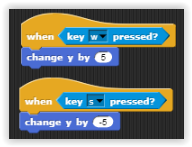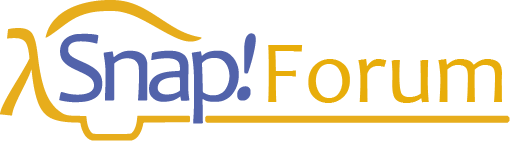# Galaxy Pong censoring bug (solved)

(Solved) If you are having problems with your pong game just look at below.

this is not a javascript forum
do you understand that concept

@toontalk shared extensive resources covering this topic.

I am confused.

Red1, Red2 must be wider to be detected when bounce.

Thank you but also sometimes it will stop when they hit the players.

The ball don't bounce on the player !

In my opinion, you do not need a true neural network machine learning AI, you just need an AI that plays pong like a human. When you play pong, you estimate where the ball is going to go on your side, then move the paddle to that spot. The AI can calculate where the ball will hit the side, then move its paddle to that spot.

But how do I make it?

something like this?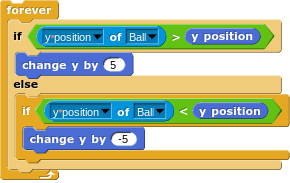replace 5 with whatever the movement meta is in your game

I made a pong AI once that calculated where the ball would land on its side. I think to do that I calculated where the ball would land on the top/bottom, then pretended the ball was there and that it bounced off the top/bottom wall, then repeated this until it detected that the ball would pass the X position of the paddle, where it would then calculate the Y value of where it would intersect with the paddle. To make the AI not actually impossible I then added this y value by a random number that got bigger the farther the ball was away from the paddle to emulate human error.

That may be confusing to read. But I think it'd be easier to understand if I drew an image.

That's what I had in mind, but I think you could just calculate at what height the ball would hit the paddle, even if that is way off the stage, then divide the y value by the stage height and take the remainder.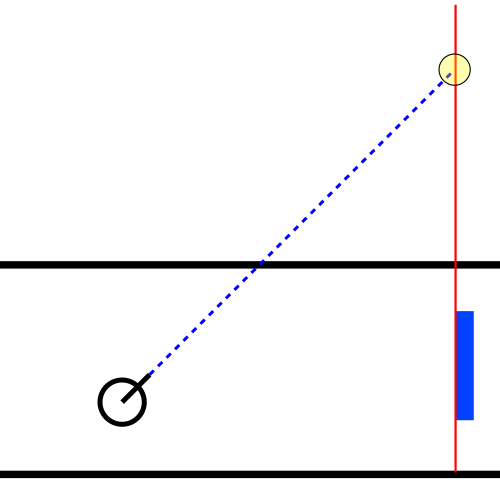No, I don't think that'd work. That'd be the same as the ball teleporting to the opposite wall when touching one. It makes a sawtooth wave. It should make a triangle wave.

Besides the Snap! blocks that I've created that build on tensorflow.js, you can use tensorflow.js yourself. There is a problem, however that manifests itself if you do anything very heavy in Tensorflow. The Snap! canvas can become corrupted so only a few elements are displayed.

I assume this is because they are competing for the GPU. You can use tensorflow.js with the CPU (while it is fast, the GPU version is much faster). The workaround I use is to run tensorflow.js in an iframe (hidden or 1 pixel in size). Then the Snap! JavaScript blocks use postMessage to get the iframe to do the real work.

This is really confusing but because Galaxy Pong isn't working I made a new 1 player one that works here is the link:

you can replace this in the code of bar (sprite(2):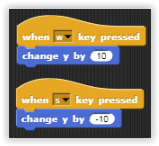by this :